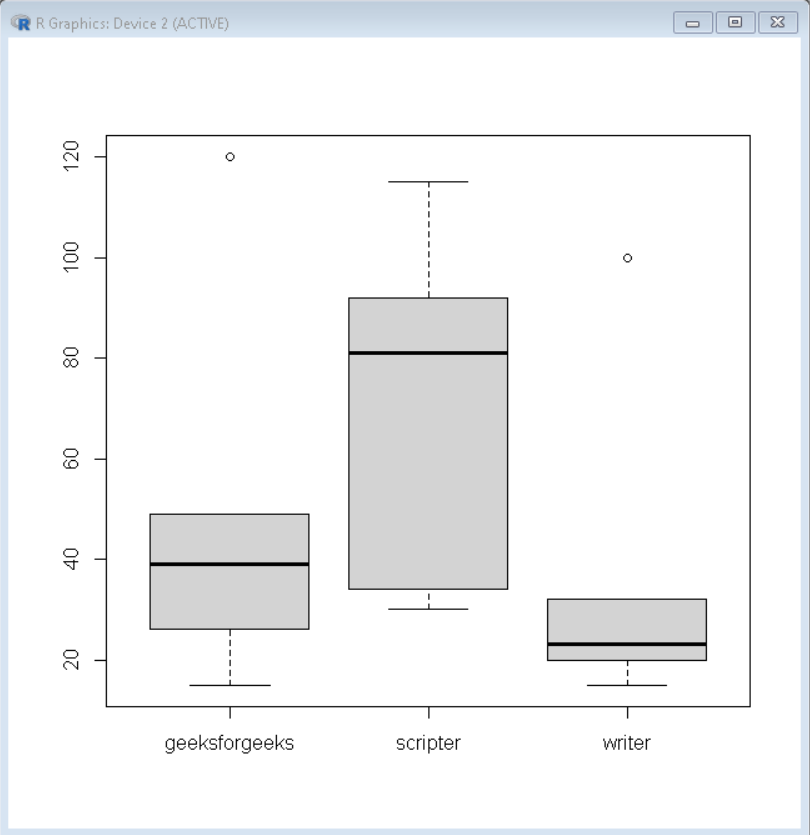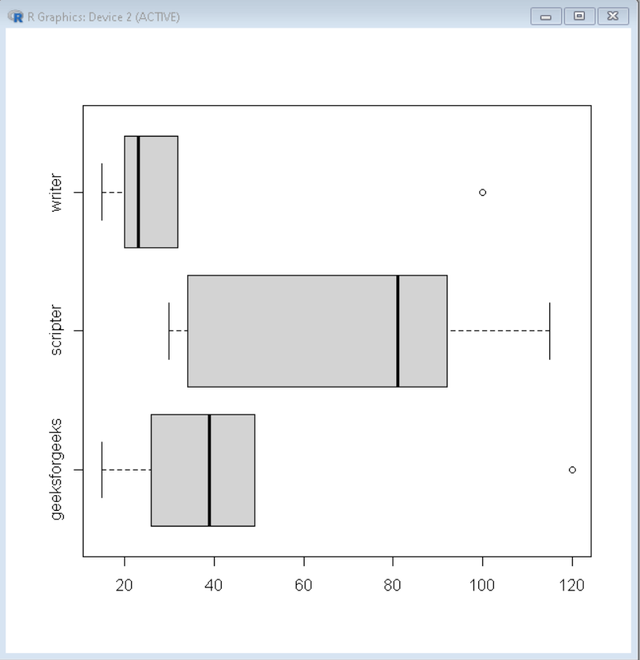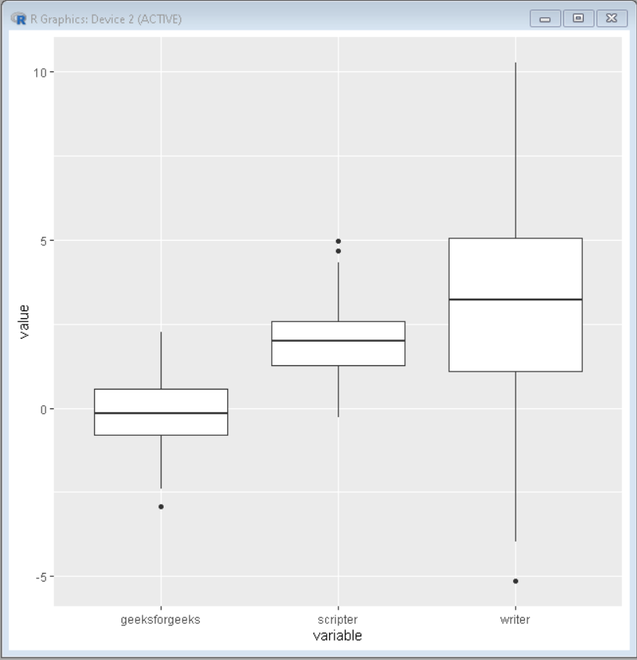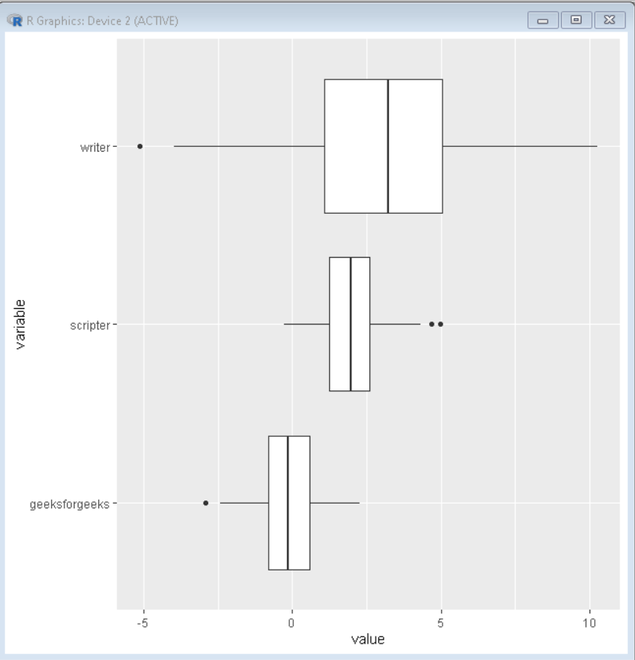Change Axis Labels of Boxplot in R

• Difficulty Level : Expert
• Last Updated : 06 Jun, 2021

A box graph is a chart that is used to display information in the form of distribution by drawing boxplots for each of them. Boxplots help us to visualize the distribution of the data by quartile and detect the presence of outliers. Adding axis labels for Boxplot will help the readability of the boxplot.

In this article, we will discuss how to change the axis labels of boxplot in R Programming Language.

Method 1: Using Base R

Boxplots are created in R Programming Language by using the boxplot() function.

Syntax:

boxplot(x, data, notch, varwidth, names, main)

Parameters:

• x: This parameter sets as a vector or a formula.
• data: This parameter sets the data frame.
• notch: This parameter is the label for horizontal axis.
• varwidth: This parameter is a logical value. Set as true to draw width of the box proportionate to the sample size.
• main: This parameter is the title of the chart.
• names: This parameter are the group labels that will be showed under each boxplot.

If made with basic R, we use the names parameter of the boxplot() function. For this boxplot data, has to be first initialized and the name which has to be added to axis is passed as vector. Then boxplot() is called with data and names parameter set to this vector.

Example:

R

 # sample data for plottinggeeksforgeeks=c(120,26,39,49,15)scripter=c(115,34,30,92,81)writer=c(100,20,15,32,23)  # labels for Axislabel=c("geeksforgeeks","scripter","writer")  # boxplot with names parameter for labelsboxplot(geeksforgeeks, scripter, writer, names=label)

Output:Boxplot with Axis Label

This can also be done to Horizontal boxplots very easily. To convert this to horizontal boxplot add parameter Horizontal=True and rest of the task remains the same. For this, labels will appear on y-axis.

Example:

R

 # sample data for plottinggeeksforgeeks=c(120,26,39,49,15)scripter=c(115,34,30,92,81)writer=c(100,20,15,32,23)  # labels for Axislabel=c("geeksforgeeks","scripter","writer")  # boxplot with names parameter for labelsboxplot(geeksforgeeks, scripter, writer,         names=label, horizontal=TRUE)

Output:Horizontal boxplot with changed labels

Method 2: Using ggplot2

If made with ggplot2, we change the label data in our dataset itself before drawing the boxplot.

Reshape module is used to convert sample data from wide format to long format and ggplot2 will be used to draw boxplot. After data is created, convert data from wide format to long format using melt function. Now, change the variable name in the dataset and simply draw the boxplot.

Example:

R

 # load package reshape2 and ggplot2library("reshape2") library("ggplot2")   # Create sample data set.seed(97364)                              sample <- data.frame(x1 = rnorm(200),                   x2 = rnorm(200, 2),                   x3 = rnorm(200, 3, 3))  # Reshape sample data to long formsample_main <- melt(sample)  # Add variable parameter for axis label in datasetlevels(sample_main\$variable) <- c("geeksforgeeks","scripter","writer")  # Draw boxplotggplot(sample_main, aes(variable, value)) + geom_boxplot()

Output:Boxplot with changed label

This can be done to Horizontal boxplots very easily. To convert this to Horizontal Boxplot add coord_flip() in the boxplot code, and rest remains the same as above.

Syntax:

geom_boxplot() + coord_flip()

Example:

R

 # load package reshape2 and ggplot2library("reshape2") library("ggplot2")   # Create sample data set.seed(97364)                              sample <- data.frame(x1 = rnorm(200),                   x2 = rnorm(200, 2),                   x3 = rnorm(200, 3, 3))  # Reshape sample data to long formsample_main <- melt(sample)  # Add variable parameter for axis label in datasetlevels(sample_main\$variable) <- c("geeksforgeeks","scripter","writer")  # Draw boxplotggplot(sample_main, aes(variable, value)) + geom_boxplot() + coord_flip()

Output:Horizontal boxplot using ggplot2 with changed label

My Personal Notes arrow_drop_up